Smartick is a fun way to learn math!Jun10

# Dividing a Decimal by a Whole Number

### Dividing a Decimal by a Whole Number

Hello everyone! In this week’s post, we are going to talk about dividing a decimal by a whole number.

You already know how to divide a whole number by a whole number by now, right? If not, check out our posts about dividing by a one-digit number here, and dividing by a two-digit number here. Once you review these lessons, we can move on to dividing a decimal by a whole!

### Dividing a decimal by a one-digit whole number

Here are steps:

1. Divide the number as you normally would, as if there was no decimal point in the number.
2. When we bring down the digit that comes after the decimal point, we have to place a decimal point in the quotient.

Example:

Let’s divide 47.5 by 5.

We are going to use normal division and start by dividing 47 by 5.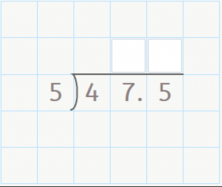47 / 5 = 9

9 x 5 = 45

47 – 45 = 2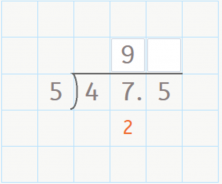Now, we have to bring down the next number, but it’s behind the decimal point.

This means that we have to place a decimal point in the quotient.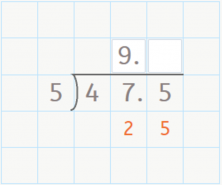Now, we divide 25 by 5.

25 / 5 = 5

25 – 25 = 0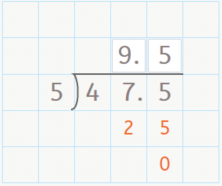We’re done dividing. The answer is 9.5

### Dividing a decimal by a two-digit whole number

These are the steps:

1 Divide the number as you would normally, as if there was no decimal point in the number.

2 When we bring down the first digit on the right side of the decimal point, we have to place a decimal point in the quotient.

Example:

Let’s divide 360.57 by 51.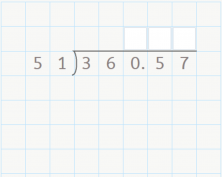360 / 51 = 7

51 x 7 = 357

360 – 357 = 3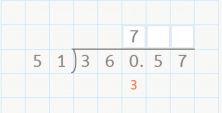Now, we are going to bring down the 5. But, because it follows the decimal point, we have to place a decimal point in the quotient.

We continue and divide 35 by 51. But 35 is less than 51, so that means we need to add a 0 in the quotient, and then we can bring down the next number.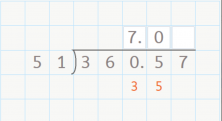Now, we’ll divide 357 by 51.

357 / 51 = 7

357 – 357 = 0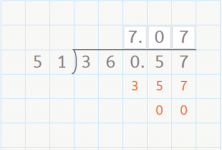We’re done dividing. The answer is 7.07